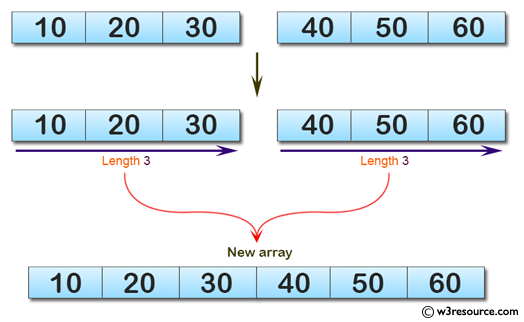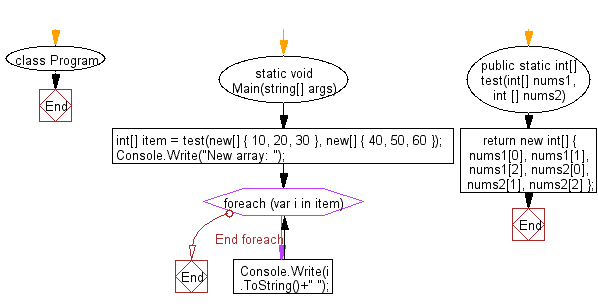﻿ C# - New array from two array of integers, each length 3# C# Sharp Basic Algorithm Exercises: Create a new array from two given array of integers, each length 3

## C# Sharp Basic Algorithm: Exercise-104 with Solution

Write a C# Sharp program to create a new array from two given array of integers, each length 3.

Pictorial Presentation:Sample Solution:-

C# Sharp Code:

``````using System;
namespace exercises
{
class Program
{
static void Main(string[] args)
{
int[] item =  test(new[] { 10, 20, 30 }, new[] { 40, 50, 60 });
Console.Write("New array: ");
foreach(var i in item)
{
Console.Write(i.ToString()+" ");
}
}
public static int[]  test(int[] nums1, int [] nums2)
{
return new int[] { nums1, nums1, nums1, nums2, nums2, nums2 };
}
}
}
```
```

Sample Output:

`New array: 10 20 30 40 50 60`

Flowchart:C# Sharp Code Editor:

Improve this sample solution and post your code through Disqus

What is the difficulty level of this exercise?

Test your Programming skills with w3resource's quiz.

﻿